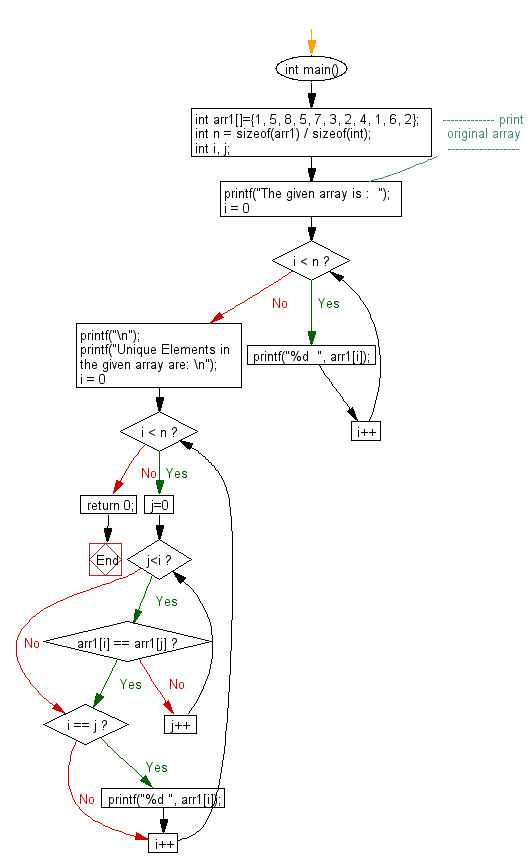﻿ C exercises: Print all unique elements of an unsorted array - w3resource# C Exercises: Print all unique elements of an unsorted array

## C Array: Exercise-73 with Solution

Write a program in C to print all unique elements of an unsorted array.

Sample Solution:

C Code:

``````#include <stdio.h>
int main()
{
int arr1[]={1, 5, 8, 5, 7, 3, 2, 4, 1, 6, 2};
int n = sizeof(arr1) / sizeof(int);
int i, j;
//------------- print original array ------------------
printf("The given array is :  ");
for(i = 0; i < n; i++)
{
printf("%d  ", arr1[i]);
}
printf("\n");
//------------------------------------------------------
printf("Unique Elements in the given array are: \n");
for(i = 0; i < n; i++)
{
for (j=0; j<i; j++)
{
if (arr1[i] == arr1[j])
break;
}

if (i == j)
{
printf("%d ", arr1[i]);
}
}
return 0;
}
```
```

Sample Output:

```The given array is :  1  5  8  5  7  3  2  4  1  6  2
Unique Elements in the given array are:
1 5 8 7 3 2 4 6
```

Flowchart:C Programming Code Editor:

Improve this sample solution and post your code through Disqus.

What is the difficulty level of this exercise?

﻿